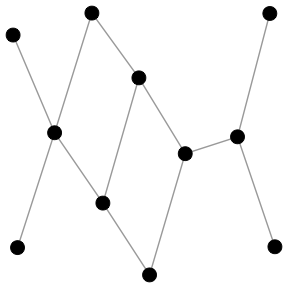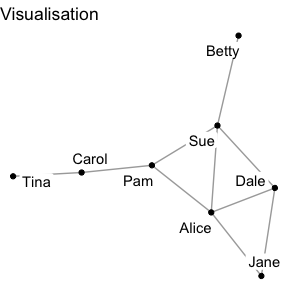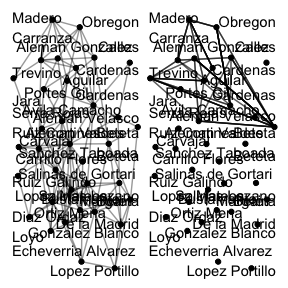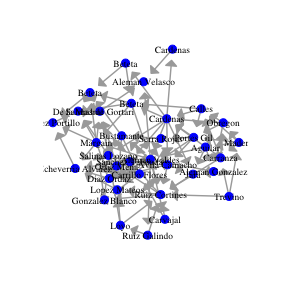# Visualising Networks

There are several packages in R for plotting and visualising network data, such as {ggraph} and {igraph}. In addition to these R packages, this vignette also introduces some functions in the {migraph} package for plotting and visualising network data in general, as well as plots that include centrality measures, plots of networks over time, and blockmodels and tree diagrams. Before covering {migraph} in more detail, we first provide a general overview of using {ggraph} and {igraph} to plot network data as {migraph} is built as an addition to both packages.

## Using {migraph}

To get a basic visualisation of the network before adding various specifications, the autographr() function in {migraph} is a quick and easy way to obtain a clear first look of the network for preliminary investigations and understanding of the network.

library(migraph)
autographr(ison_brandes)We can also specify the colours, groups, shapes, and sizes of nodes in the autographr() function using the following parameters:

• node_colour

• node_shape

• node_size

Append ggtitle() from the {ggplot2} package to add a title. {migraph} works well with both {ggplot2} and {ggraph} functions that can be appended to create more tailored visualisations of the network.

library(migraph)
labels = TRUE,
node_size = 1.5) +
ggplot2::ggtitle("Visualisation")### Visualising networks over time

The gglineage(), ggevolution(), and ggatyear() functions can be used to visualise the changes in the network over time. gglineage() shows how observations are connected to preceding or succeeding observations. ggevolution() plots two networks for comparison of the changes in their structure from one to the next. ggatyear() selects and plots a network at the specified point in time.

library(migraph)
mpn_elite_mex2 <- mpn_elite_mex  %>%
tidygraph::activate(edges) %>%
tidygraph::reroute(from = sample.int(11, 44, replace = TRUE),
to = sample.int(11, 44, replace = TRUE))
ggevolution(mpn_elite_mex, mpn_elite_mex2)## Using {ggraph}

For more flexibility with visualizations, {migraph} users are encouraged to use the excellent {ggraph} package. {ggraph} is built upon the venerable {ggplot2} package and works with tbl_graph and igraph objects. As with {ggplot2}, {ggraph} users are expected to build a particular plot from the ground up, adding explicit layers to visualise the nodes and edges.

library(ggraph)
ggraph(mpn_elite_mex, layout = "fr") +
arrow = arrow(angle = 45,
length = unit(2, "mm"),
type = "closed"),
end_cap = circle(3, "mm")) +
geom_node_point(size = 2.5, shape = 19, colour = "blue") +
geom_node_text(aes(label=name), family = "serif", size = 2.5) +
scale_edge_width(range = c(0.3,1.5)) +
theme_graph() +
theme(legend.position = "none")As we can see in the code above, we can specify various aspects of the plot to tailor it to our network. Firstly, we can alter the layout of the network using the layout = argument to create a clearer visualisation of the ties between nodes. This is especially important for larger networks, where nodes and ties are more easily obscured or misrepresented. In {ggraph}, the default layout is the “stress” layout. The “stress” layout is a safe choice because it is deterministic and fits well with almost any graph, but it is also a good idea to explore and try out other layouts on your data. More layouts can be found in the {graphlayouts} and {igraph} R packages. To use a layout from the {igraph} package, enter only the last part of the layout algorithm name (eg. layout = "mds" for “layout_with_mds”).

Secondly, using geom_node_point() which draws the nodes as geometric shapes (circles, squares, or triangles), we can specify the presentation of nodes in the network in terms of their shape (shape=, choose from 1 to 21), size (size=), or colour (colour=). We can also use aes() to match to node attributes. To add labels, use geom_node_text() or geom_node_label() (draws labels within a box). The font (family=), font size (size=), and colour (colour=) of the labels can be specified.

Thirdly, we can also specify the presentation of edges in the network. To draw edges, we use geom_edge_link0() or geom_edge_link(). Using the latter function makes it possible to draw a straight line with a gradient. The following features can be tailored either globally or matched to specific edge attributes using aes():

• colour: edge_colour=

• width: edge_width=

• linetype: edge_linetype=

• opacity: edge_alpha=

For directed graphs, arrows can be drawn using the arrow= argument and the arrow() function from {ggplot2}. The angle, length, arrowhead type, and padding between the arrowhead and the node can also be specified.

To change the position of the legend, add the theme() function from {ggplot2}. The legend can be positioned at the top, bottom, left, or right, or removed using “none”.